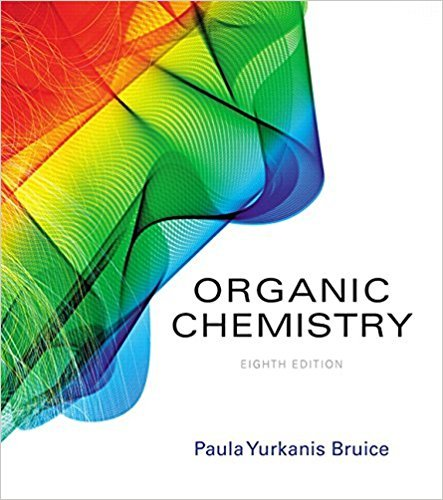×
×

# Solutions for Chapter 7.12: An Introduction to Multistep Synthesis## Full solutions for Organic Chemistry | 8th Edition

ISBN: 9780134042282Solutions for Chapter 7.12: An Introduction to Multistep Synthesis

Solutions for Chapter 7.12
4 5 0 249 Reviews
12
0
##### ISBN: 9780134042282

Organic Chemistry was written by and is associated to the ISBN: 9780134042282. This textbook survival guide was created for the textbook: Organic Chemistry, edition: 8. This expansive textbook survival guide covers the following chapters and their solutions. Chapter 7.12: An Introduction to Multistep Synthesis includes 15 full step-by-step solutions. Since 15 problems in chapter 7.12: An Introduction to Multistep Synthesis have been answered, more than 31108 students have viewed full step-by-step solutions from this chapter.

Key Chemistry Terms and definitions covered in this textbook
• a-Hydrogen

A hydrogen on a carbon alpha to a carbonyl group.

A reaction in which two atoms or groups of atoms react with a double bond, forming a compound with the two new groups bonded to the carbons of the original double bond.

• amine

Compounds containing a nitrogen atom that is connected to one, two, or three alkyl or aryl groups.

• chemical energy.

Energy stored within the structural units of chemical substances. (6.1)

• chemical equilibrium

A state of dynamic balance in which the rate of formation of the products of a reaction from the reactants equals the rate of formation of the reactants from the products; at equilibrium the concentrations of the reactants and products remain constant. (Section 4.1;Chapter 15: Introduction)

• delocalized molecular orbitals.

Molecular orbitals that are not confined between two adjacent bonding atoms but actually extend over three or more atoms. (10.8)

• diffusion.

The gradual mixing of molecules of one gas with the molecules of another by virtue of their kinetic properties. (5.7)

• divalent

An element that forms two bonds, such as oxygen.

• equilibrium-constant expression

The expression that describes the relationship among the concentrations (or partial pressures) of the substances present in a system at equilibrium. The numerator is obtained by multiplying the concentrations of the substances on the product side of the equation, each raised to a power equal to its coefficient in the chemical equation. The denominator similarly contains the concentrations of the substances on the reactant side of the equation. (Section 15.2)

• Friedel-Crafts alkylation

An electrophilic aromatic substitution reaction that installs an alkyl group on an aromatic ring.

• high-resolution mass spectrometry

A technique that involves the use of a detector that can measure the m/z values to four decimal places.This technique allows for the determination of the molecular formula of an unknown compound.

• intermediate

A structure corresponding to a local minimum (valley) in an energy diagram.

• Meisenheimer complex

Theresonance-stabilized intermediate of a nucleophilicaromatic substitution reaction.

• Melt transition (Tm)

The temperature at which crystalline regions of a polymer melt.

• oxime

A compound with the structure R2CRN!OH.

• oxirane

A cyclic ether containing a three-membered ring system. Also called an epoxide.

• Plane of symmetry

An imaginary plane passing through an object dividing it so that one half is the mirror image of the other half

• retention time

The amount of time required for a compound to exit from a gas chromatograph.

• saponification

Hydrolysis of an ester in the presence of a base. (Section 24.4)

• vicinal

A term used to describe two identical groups attached to adjacent carbon atoms.

×